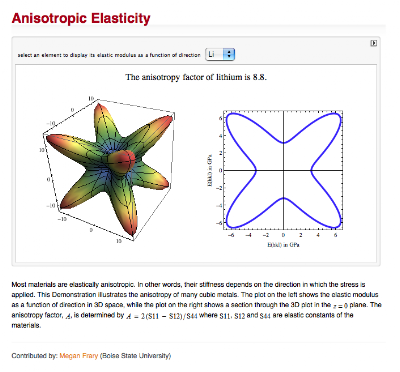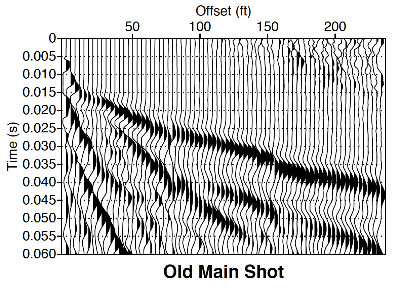## Sunday, December 1, 2013

### Refraction interpretation with Mathematica

Don’t ask me for help unless you have Googled it first.  Anonymous

--------------------------

My last Seismos column in The Leading Edge was a slightly-modified version of my Mathematica Strat Column blog entry. It showed that an interactive stratigraphic column could be made using the Mathematica Manipulate function.  At the SEG meeting in Houston last week, I spoke a minute with Jim Gaiser who was discovering all the great things that Manipulate can do.

It made me think that a little discussion would be useful.  Manipulate has the full power of M’s analytical, numerical, and graphical behind it, and allows you to set parameters via slider bars or other kinds of controllers.  This may not sound like much, but it is immensely powerful.

Consider for example an equation with one coordinate (x) and 4 parameters (a,b,c,d):   y = a + b x + c x^2 + d x^3.  Given numerical values for (a,b,c,d) we can make a plot in any graphics system, or we can fix (a,b,c) and plot a family of curves associated with various values of d.  Or we could let, say, c be the parameter that takes on various values, giving another family of curves. It would also be useful to make a 3D plot of the equation with axes of x and one of the parameters, or maybe even a 3D surface movie as we cycle through a second parameter.  But none of these methods really lets us figure out the effect of all 4 parameters. Manipulate let's us make a single plot with default values for (a,b,c,d) and slider bars to play around with other values of these parameters.  Again it sounds insignificant, but it is not.  What we are actually doing is exploring a multidimensional parameter space.  Our example has 5 parameters, but there could be 10, 20, or more.  Clearly when you get into the hundreds or thousands of parameters, an interactive tool like Manipulate will cease to be useful. But a universe of problems have 15 parameters or less, including important things like Gassmann fluid substitution.

For several years, Wolfram Research (the makers of M) have maintained a contributed site of Manipulate examples from M users around the world.  The site is called The Wolfram Demonstrations Project (Google it).  To get started, look for “Weibull Statistics for Fracture Data” or “Anisotropic Elasticity” (see Figure 1). If you have an M license, the Demonstrations site is a gold mine of source code.  Without a license, a demonstration will cycle through parameter selections.  To get full manipulation capability for demonstrations in a browser window without an M license, just download the free Wolfram CDF Player.  CDF (computable document format) is analogous to the PDF format, but allows results to be recomputed.  This is a step toward reproducible research, a subject of long interest to Stanford’s Jon Claerbout (Google Claerbout reproducible research).

Manipulate can be very useful for interpretation.  To give one example, consider the zero-dip 4-layer refraction problem.  The theory behind this problem can be found in many standard texts (e.g., Telford et al., 1976, p.278-281). The equations give travel time as a function of offset for the direct wave and refraction arrivals from base of layers 2, 3, and 4.  The equations are rather complicated and depend on several model parameters, including thickness and velocity of each layer, the critical angles, etc.  Someone good in math can get it pretty quickly, but my geologists would struggle to see how it works and, more importantly, how it relates to field data.

In August of 2013, we did a trial seismic experiment (Figure 2) on the lawn of Old Main, the original 1871 building at the University of Arkansas.  Well, I went back to that data and manually picked first breaks using SeismicUnix, creating a list of (time,offset) pairs that were converted to CSV format in a spreadsheet.  Bringing this into M, allows us to make a Manipulate widget that overlays theoretical refraction travel time curves on the observed data points.  Geology students can then adjust model parameters until an acceptable fit is achieved (Figure 3).

In other words, we are doing 7-parameter manual inversion using M’s Manipulate function. Unlike automatic inversion, students gain an understanding of the role played by various parameters and can get results despite minor data problems.  On the latter topic, note there is a section of time-shifted data between 80 and 150 ft, likely a trace header geometry bust.  No problem, with this intuitive interface and display we can work around the time shift, and fit the suspect interval parallel to measured data.

From state survey geological maps and various building excavations, it is known that Pennsylvanian shale and sandstone lie below the soil layer at this site and deeper Miss. Fayetteville shale is found in outcrop 80 ft down the hill. At some depth beneath our test site lies Pitkin limestone representing the major Miss/Penn unconformity.  Such is the knowledge from 140 years of surface geology. With this small seismic experiment we can now estimate Pitkin at 52 ft below the lawn of Old Main. Ya gotta love geophysics.Figure 1. Screen shot of Megan Fray’s elastic anisotropy Manipulate example on the Wolfram Demonstrations Project web site. The 3D plot can be interactively rotated for better inspection.Figure 2. Early arrival plot of hammer-source field seismic data from U Arkansas campus.Figure 3. Interactive 4-layer refraction interpretation using Mathematica Manipulate function, showing picked first arrivals (red dots) and theoretical travel time curves (lines).